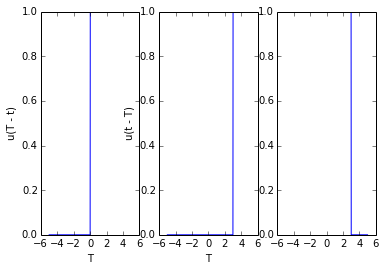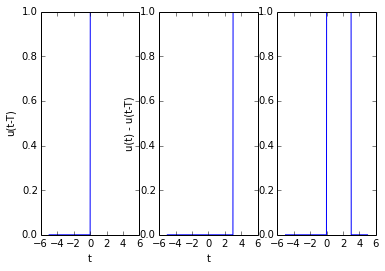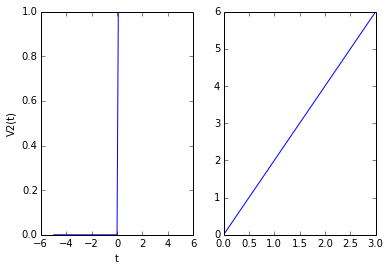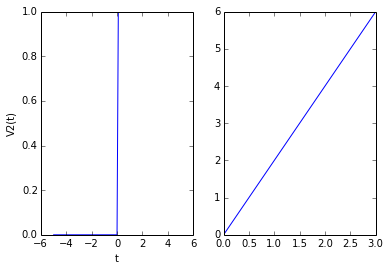# Chapter 1 : Introduction of Signals and Spectra¶

## Example 1.1 Page No : 12¶

In :
import math
#page 12
#problem 1.1

#Given signal u  =  2*math.exp(-3*t)

#Since the function integral does not accept %inf as limit we need to use approximation by changing variables.

#First the signal is to be expressed in terms of 'x'.

def Signal(x):
return 2*math.exp(-3*x);

#We then substitute x  =  math.tan(z), and then express the given signal wrt 'z' and not 'x'.

def Gmodified(z):
x = math.tan(z);
return (Signal(x))**2/(math.cos(z))**2;

# Results
print 'The energy of this signal is %.3f'%E

The energy of this signal is 0.667


## Example 1.2 Page No : 12¶

In :
import math

#Given signal u  =  2*math.sin(0.5*math.pi*t)

#Since u is periodic, averaging over -infinity to + infinity will give the same result as averaging over -2 to 2, where 4 is the time period.
t0  =  -2;
t1  =  2;

# Calculations
def f1(t):
return (2*math.sin(0.5*math.pi*t))**2

# Results
print 'The power of the signal is ',E

The power of the signal is  8.0


## Example 1.3 Page No : 18¶

In :
%matplotlib inline
from matplotlib.pyplot import plot,subplot,xlabel,ylabel
import math
from numpy import arange,zeros
#page 18
#problem 1.3

#u1(T) vs T
T  =  arange(-5,5+0.0082,0.0082)
u1 = zeros(len(T))
u1[T <= 0]  =  0;
u1[T>0]  =  1;
xlabel('T');
ylabel('u(T)')

subplot(131);
plot(T,u1);

#u2(T-t) vs T
#Shifting the given signal by t units to the right, we get
#Let us assume the amount of time to be shited is 3 units
t  =  3;

T  =  arange(-5,5+0.0082,0.0082)
u2 = zeros(len(T))
u2[T<= t]  =  0;
u2[T>t]  =  1;
xlabel('T');
ylabel('u(T - t)')

subplot(132);
plot(T,u2);

#u(t - T)  =  u(-(T - t))

T  =  arange(-5,5+0.0082,0.0082)
u3 = zeros(len(T))
u3[T>= t]  =  0;
u3[T<t]  =  1;
xlabel('T');
ylabel('u(t - T)')

subplot(133);
plot(T,u3);## Example 1.4 Page No : 18¶

In :
%matplotlib inline
from matplotlib.pyplot import plot,subplot,xlabel,ylabel
import math
from numpy import arange,zeros

#u1(t)
t  =  arange(-5,5+0.0082,0.0082)
u1 = zeros(len(t))
u1[t<= 0]  =  0;
u1[t>0]  =  1;

xlabel('t');
ylabel('u(t)')

subplot(131);
plot(t,u1);

#u2(t-T)
#Shifting the given signal by t units to the right, we get
#Let us assume the amount of time to be shited is 3 units
T  =  3;

t  =  arange(-5,5+0.0082,0.0082)
u2 = zeros(len(t))
u2[t<= T]  =  0;
u2[t>T]  =  1;

xlabel('t');
ylabel('u(t-T)')

subplot(132);
plot(t,u2);

#u(t) - u(t - T)

t  =  arange(-5,5+0.0082,0.0082)
u3  =  u1 - u2;

xlabel('t');
ylabel('u(t) - u(t-T)')

subplot(133);
plot(t,u3);## Example 1.5 Page No : 18¶

In :
%matplotlib inline
import math
from numpy import arange,zeros
from matplotlib.pyplot import subplot,xlabel,ylabel,plot
#page 18
#problem 1.5

#V1(t)  =  u(t) - u(t - 5)
t  =  arange(-5,5+0.1,0.1)
V1 = zeros(len(t))
V1[t<= 0]  =  0;
V1[t>0]  =  1;

xlabel('t');
ylabel('V1(t)')
subplot(121);
plot(t,V1);

#V2(t)  =  2*t*(u(t) - u(t - 3))
t  =  arange(0,3+0.1,3);
V2  =  2*t;

xlabel('t');
ylabel('V2(t)')
subplot(122);
plot(t,V2);

#Autocorrelation R12(0)  =  R

def f2(t):
return 2*t

def f3(t):
return 1

#In the textbook, E2 has been computed as 18 instead of 36

def f4(t):
return 4*t**2

c  =  R/(E1*E2)**0.5;

print 'The correlation term R12(0) is ',R
print 'The autocorrelation term R1(0) is ',E1;
print 'The autocorrelation term R2(0) is ',E2;

The correlation term R12(0) is  9.0
The autocorrelation term R1(0) is  5.0
The autocorrelation term R2(0) is  36.0## Example 1.6 Page No : 19¶

In :
%matplotlib inline
import math
from numpy import arange,zeros
from matplotlib.pyplot import subplot,xlabel,ylabel,plot

#V1(t)  =  u(t) - u(t - 5)
t  =  arange(-5,5+0.1,.1)
V1 = zeros(len(t))
V1[t<= 0]  =  0;
V1[t>0]  =  1;

xlabel('t');
ylabel('V1(t)')
subplot(121);
plot(t,V1);

#V2(t)  =  2*t*(u(t) - u(t - 3))
t  =  arange(0,3+0.1,.1)
V2  =  2*t;

xlabel('t');
ylabel('V2(t)')
subplot(122);
plot(t,V2);

#Autocorrelation R12(1)  =  Ra
#The range is t  =  0 to 2, as signal V2(t) has been shifted left by one unit, V2(t-1)

def f5(t):
return 2*(t+1)

print 'The correlation term R12(1) is ',Ra

#Autocorrelation R12(-1)  =  Rb
#The range is t  =  1 to 4, as signal V2(t) has been shifted right by one unit, V2(t+1)

def f6(t):
return 2*(t-1)


The correlation term R12(1) is  8.0